# 蓝桥杯之贪心

### 蓝桥杯之贪心

• 1055.股票买卖II
• 104.货仓选址
• AcWing112.雷达设备
• 1235.付账问题
• 1239.乘积最大
• K是奇数，需要转化为K是偶数的情况，于是先取一个数，为了使得结果最大，取最大的数（正数的话绝对值最大，负数的话(K是奇数且最大值是负数，res一定是负数），最大的负数绝对值最小也能使得res尽可能大
• 转化为选取偶数的情况，将n个数两两合并后，取 K/2个数。怎么进行合并，首先如果n是偶数，两两合并正好，n若是奇数，剩下的一个数可能是正数(n中有奇数个正数），不进行合并，剩下的一个数可能是负数(n中有奇数个负数），取K/2个数时直接略过这个负数
• 为什么要从左右两端开始两两合并？因为最大值只可能出现在两段。最右端如果俩正数，最左端如果俩负数，相乘得到的绝对值是尽可能大的(sign是考虑到是否一开始就乘了一个负数），因为数组是经过排序的，绝对值最小的数一定在两段被排除的一端或者是中间（绝对值的乘积是最小的），因此只要考虑两边得到绝对值尽可能大的乘积，中间可以直接不考虑
• 注意取余顺序—— res%mod，res先取余是没有意义的，res%mod*lt计算到这里时就已经爆longlong了

## 1055.股票买卖II

#include <iostream>
using namespace std;
#define int long long int
const int N=105;
int n,x;

signed main(){
cin>>n;
int res=0;
for(int i=1;i<=n;i++){//多次买卖一支股票
cin>>x;
}

}
cout<<res;
return 0;
}


## 104.货仓选址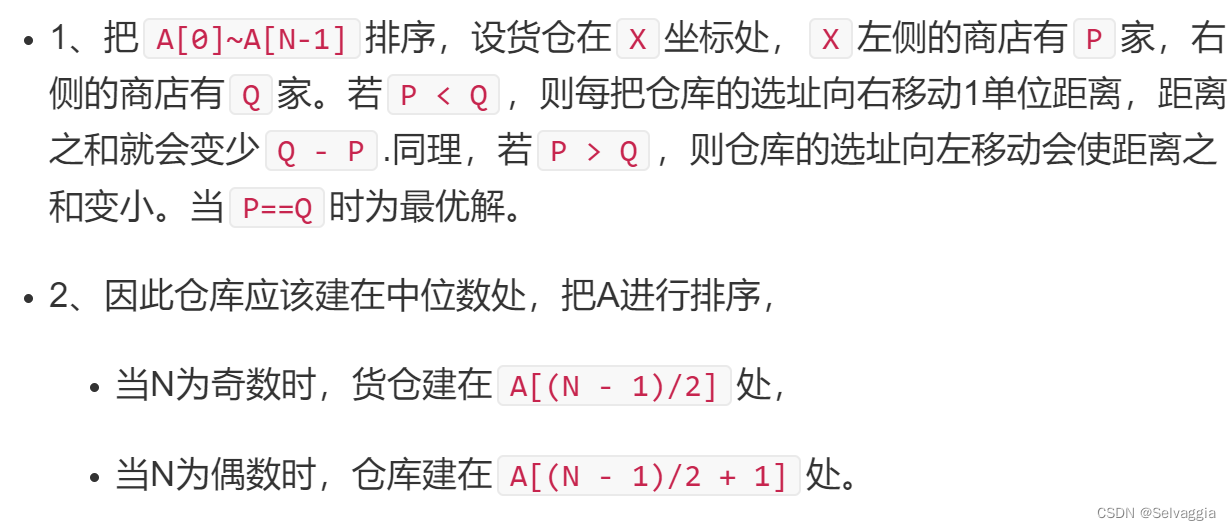#include <bits/stdc++.h>
using namespace std;
#define int long long int
const int N=1e5+5;
int n,x;
int a[N];
signed main(){
cin>>n;
int res=0;
int mid=(n+1)/2;// 1 2 3 ，1 2 3 4
for(int i=1;i<=n;i++){//货仓应该建在 第n+1 /2 个商店处
cin>>a[i];
}
sort(a+1,a+n+1);
for(int i=1;i<=n;i++){//货仓应该建在 第n+1 /2 个商店处

res+=abs(a[mid]-a[i]);

}
cout<<res;
return 0;
}



## AcWing112.雷达设备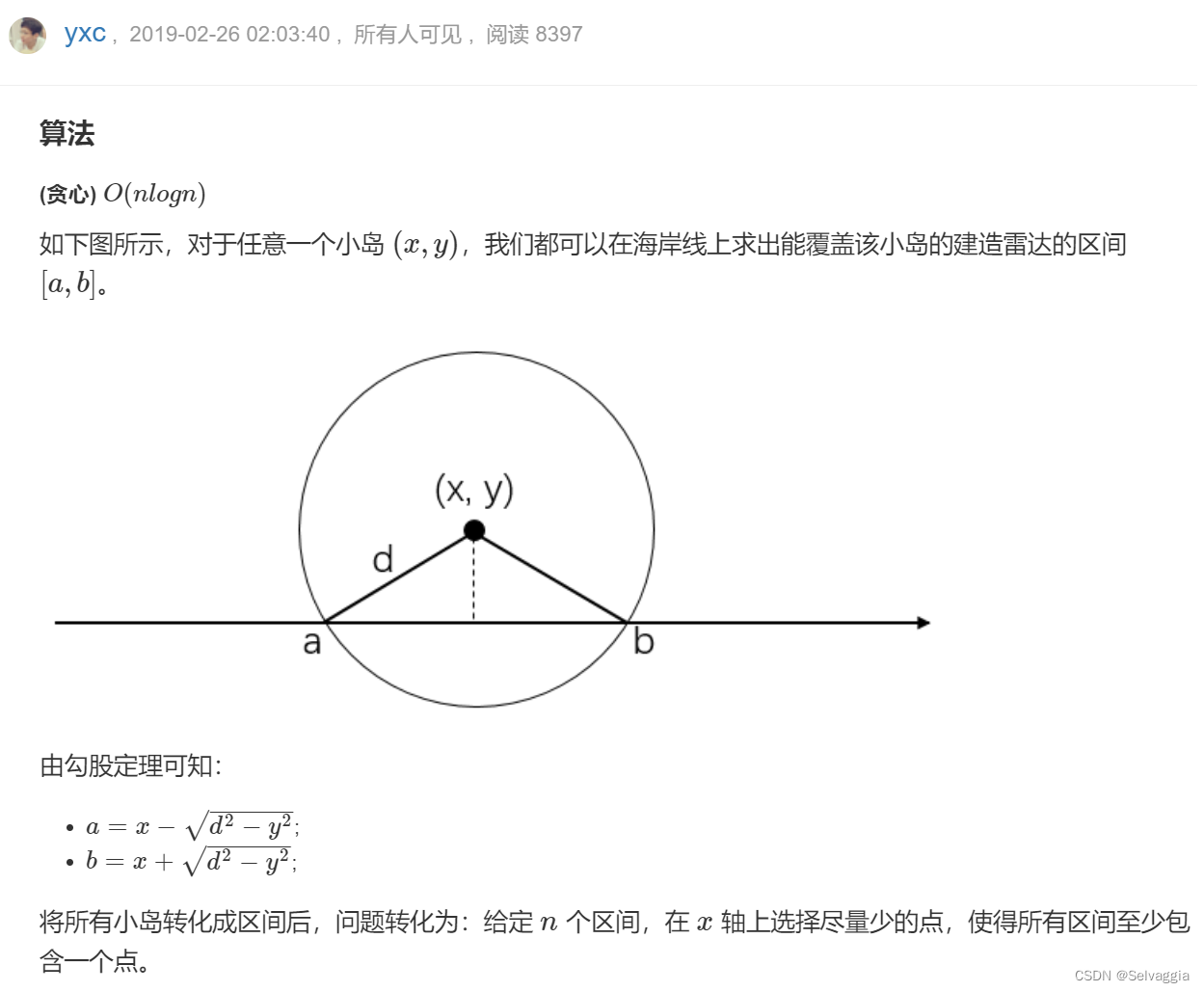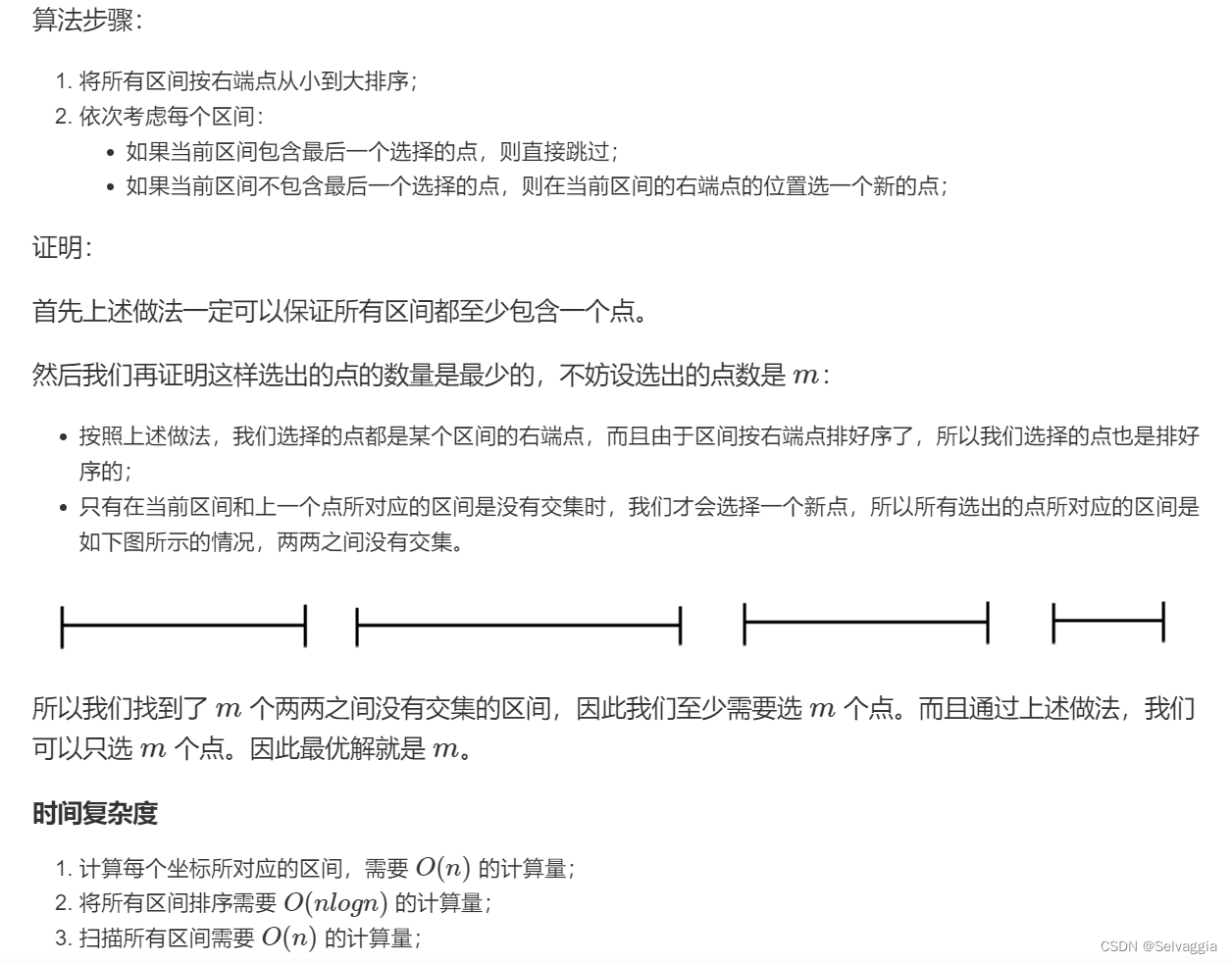• 根据右端点排序
• 注意点，node类型，每个区间段的左右端点必须精确到double，还有上一个取点的位置，也必须精确到double
#include <bits/stdc++.h>
using namespace std;
#define int long long int

const int N=1e5+5;
int n,d;

struct node{
double l,r;
bool operator<(const node & a)const{
return r<a.r;
}
}range[N];
signed main(){//雷达装置均位于海岸线上
cin>>n>>d;
int x,y;
int flag=false;
for(int i=1;i<=n;i++){
cin>>x>>y;
if(y>d){
flag=true;
cout<<"-1";
return 0;
}
range[i].l=x-sqrt(d*d-y*y);
range[i].r=x+sqrt(d*d-y*y);

}//要想覆盖这个岛，雷达的x坐标范围是[x-sqrt(d^2-y^2),x+(d^2-y^2)]
if(flag){
cout<<"-1";
return 0;
}
sort(range+1,range+n+1);
int res=0;
double pre=-2000;//上一个取点的位置
// 要求取尽可能少的点，使得每个区间段range[i]都包含至少一个点
// 那么，对于每个区间。如果上个取点如果落在这个区间，这个区间不取，否则，在这个区间的右端点取新的点
for(int i=1;i<=n;i++){//货仓应该建在 第n+1 /2 个商店处
if(pre<range[i].l){
res++;
pre=range[i].r;
}
}
cout<<res;
return 0;
}



pair自动排序

#include <bits/stdc++.h>
using namespace std;
#define int long long int
#define pdd pair<double,double>
const int N=1e5+5;
int n,d;
// struct node{
//     double l,r;
//     bool operator<(const node & a)const{
//         return r<a.r;
//     }
// }range[N];
pdd range[N];
signed main(){//雷达装置均位于海岸线上
cin>>n>>d;
int x,y;
int flag=false;
for(int i=1;i<=n;i++){
cin>>x>>y;
if(y>d){
flag=true;
cout<<"-1";
return 0;
}
// range[i].l=x-sqrt((double)d*d-y*y);
// range[i].r=x+sqrt((double)d*d-y*y);
range[i]={x+sqrt((double)d*d-y*y),x-sqrt((double)d*d-y*y)};

}//要想覆盖这个岛，雷达的x坐标范围是[x-sqrt(d^2-y^2),x+(d^2-y^2)]
if(flag){
cout<<"-1";
return 0;
}
sort(range+1,range+n+1);
int res=0;
double pre=-20000000;//上一个取点的位置
// 要求取尽可能少的点，使得每个区间段range[i]都包含至少一个点
// 那么，对于每个区间。如果上个取点如果落在这个区间，这个区间不取，否则，在这个区间的右端点取新的点
for(int i=1;i<=n;i++){//货仓应该建在 第n+1 /2 个商店处
if(pre+1e-5<range[i].second){
res++;
pre=range[i].first;
}
}
cout<<res;
return 0;
}



## 1235.付账问题

#include <bits/stdc++.h>
using namespace std;
#define int long long int

const int N=5e5+5;
int n,s;
int a[N];

signed main(){
cin>>n>>s;
int x,y;
double res;
double AVG=1.0*s/n;//此时的平均值
for(int i=1;i<=n;i++){
cin>>a[i];
}

sort(a+1,a+n+1);
double avg=AVG;
for(int i=1;i<=n;i++){
if(a[i]<avg){//钱不够的人只能出所有的钱，而且一旦出现这种情况，后面的人需要出的钱
res+=(a[i]-AVG)*(a[i]-AVG);
s-=a[i];
avg=1.0*s/(n-i);
}
else{//每个人出的钱要尽可能相同，钱不够没办法只能出全部，钱多的人要出一样的钱 来填满总额
s=0;
res+=(avg-AVG)*(avg-AVG)*(n-i+1);
break;
}
}
res/=n;
res=sqrt(res);

cout<<fixed<<setprecision(4)<<res;
return 0;
}



## 1239.乘积最大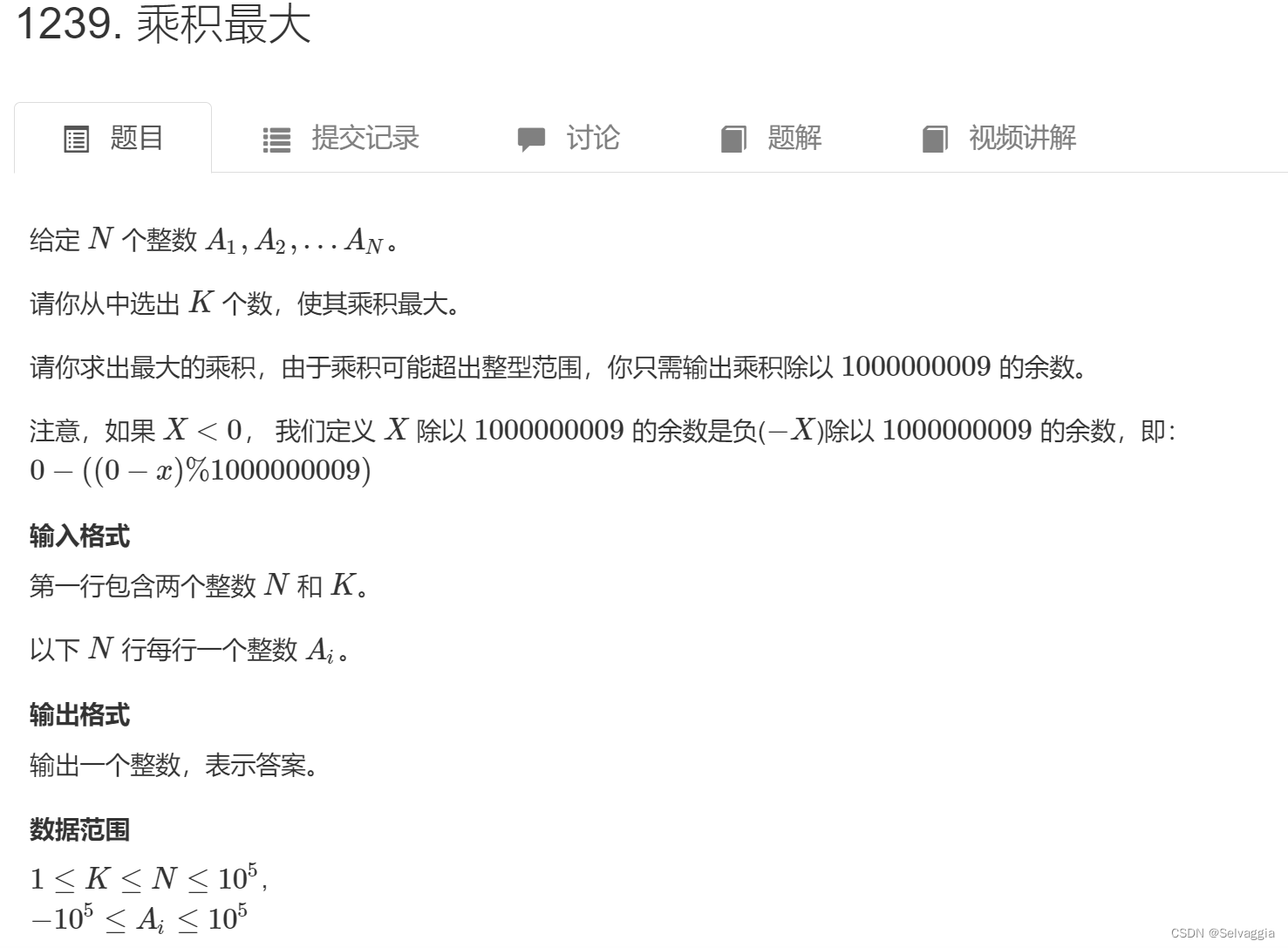5 3
-100000
-10000
2
100000
10000


999100009


5 3
-100000
-100000
-2
-100000
-100000


-999999829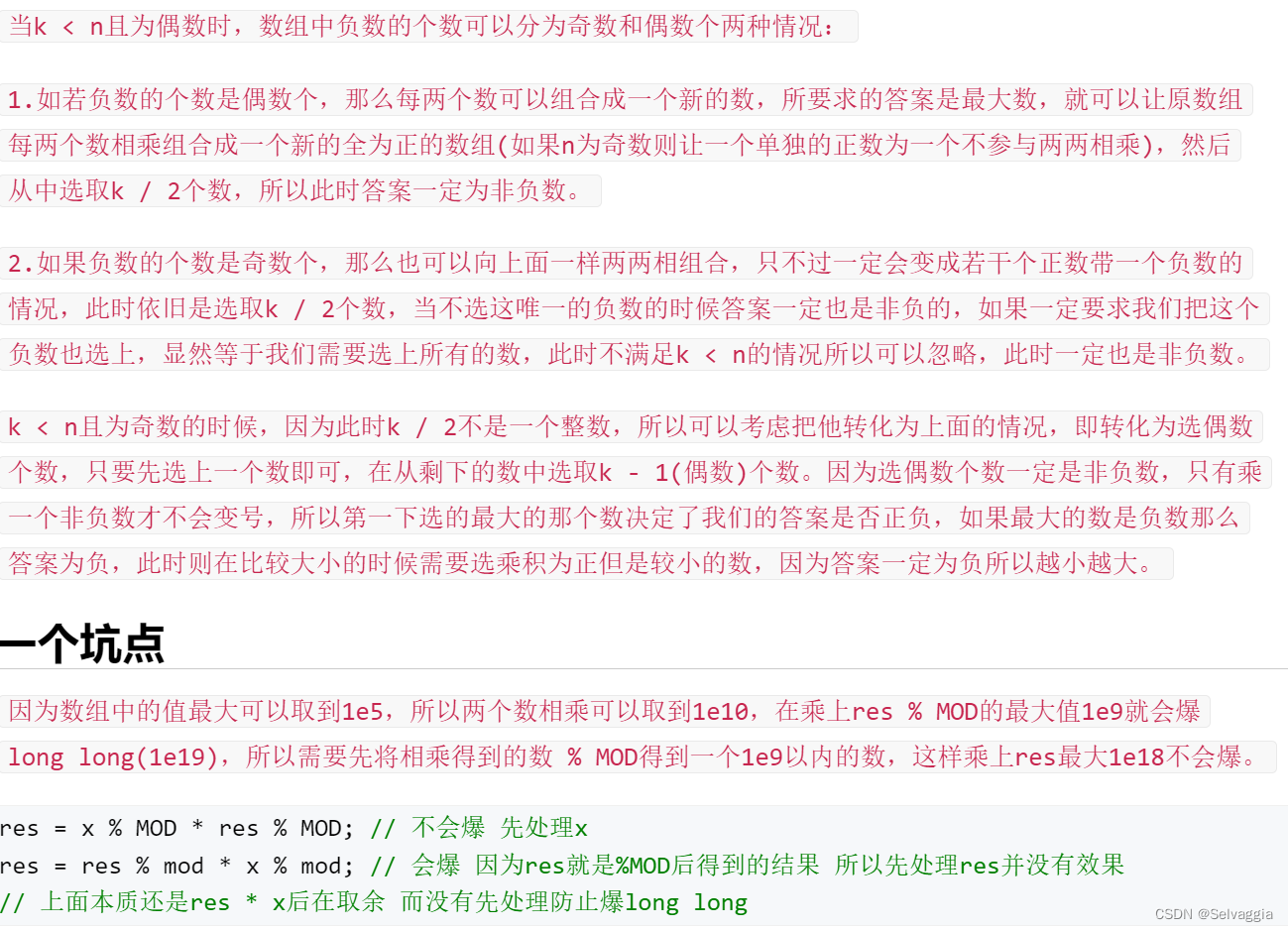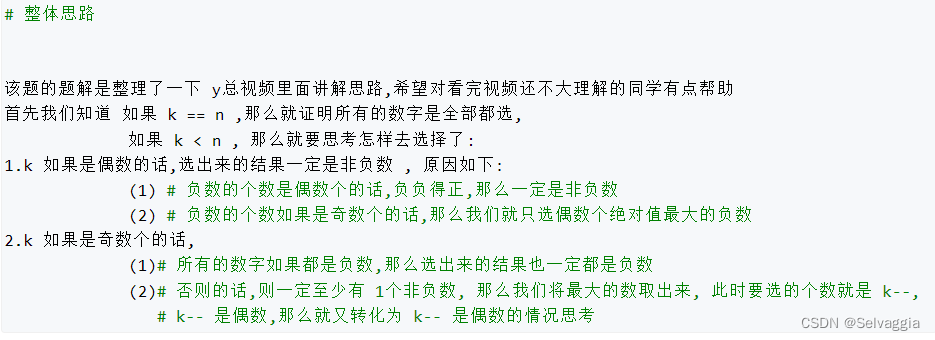### 注意取余顺序—— res%mod，res先取余是没有意义的，res%mod*lt计算到这里时就已经爆longlong了

 res=lt%mod*res%mod; ✔
//   res=res%mod*lt%mod;❌

#include <bits/stdc++.h>
using namespace std;
#define int long long int

// const int mod=1000000009;
// const int N=1e5+5;
const int N = 100010 , mod = 1000000009 ;
int n,k;
int a[N];

signed main()
{
cin>>n>>k;
for(int i=1;i<=n;i++){
cin>>a[i];
}
sort(a+1,a+1+n);
int res=1;
int sign=1;
int l=1,r=n;
if(k&1){//如果k是奇数，先取走一个，转化为k是偶数的情况
res=a[r];
if(res<0)sign=-1;
r--;
k--;
}
while(k){
int lt=a[l]*a[l+1];
int rt=a[r]*a[r-1];
if(lt*sign>rt*sign){
l+=2;
res=lt%mod*res%mod;
//   res=res%mod*lt%mod;
}
//   因为x最大是 10^10，如果不先取模的话，和res相乘的结果最大是 10^19,会暴long long
else {
r-=2;
//   res=res%mod*rt%mod;
res=rt%mod*res%mod;
}
k-=2;
}
cout<<res;

return 0;
}